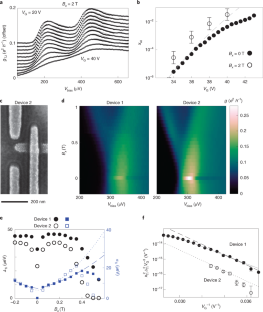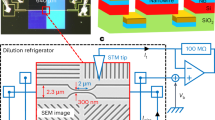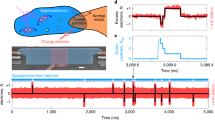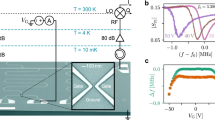Thank you for visiting nature.com. You are using a browser version with limited support for CSS. To obtain the best experience, we recommend you use a more up to date browser (or turn off compatibility mode in Internet Explorer). In the meantime, to ensure continued support, we are displaying the site without styles and JavaScript.

# High-energy quasiparticle injection into mesoscopic superconductors

## Abstract

At non-zero temperatures, superconductors contain excitations known as Bogoliubov quasiparticles (QPs). The mesoscopic dynamics of QPs inform the design of quantum information processors, among other devices. Knowledge of these dynamics stems from experiments in which QPs are injected in a controlled fashion, typically at energies comparable to the pairing energy1,2,3,4,5. Here we perform tunnel spectroscopy of a mesoscopic superconductor under high electric fields. We observe QP injection due to field-emitted electrons with 106 times the pairing energy, an unexplored regime of QP dynamics. Upon application of a gate voltage, the QP injection decreases the critical current and, at sufficiently high electric field, a field-emission current (<0.1 nA in our device) switches the mesoscopic superconductor into the normal state, consistent with earlier observations6. We expect that high-energy injection will be useful for developing QP-tolerant quantum information processors, will allow rapid control of resonator quality factors and will enable the design of electric-field-controlled superconducting devices with new functionality.

This is a preview of subscription content, access via your institution

## Access options

Prices vary by article type

from$1.95 to$39.95

Prices may be subject to local taxes which are calculated during checkoutFig. 1: Tunnel spectroscopy and critical current of a titanium nanowire at high gate voltage (VG) and 20 mK temperature.Fig. 3: Injection in fixed high magnetic field, a second device and a model of QP injection due to field emission.

### Similar content being viewed by others### Thermalization and dynamics of high-energy quasiparticles in a superconducting nanowire

T. Jalabert, E. F. C. Driessen, … C. Chapelier### A superconductor free of quasiparticles for seconds

E. T. Mannila, P. Samuelsson, … J. P. Pekola### On the origin of the controversial electrostatic field effect in superconductors

I. Golokolenov, A. Guthrie, … V. Tsepelin

## Data availability

The transport data and device images that support the findings of this study are publicly available in the Harvard Dataverse with the identifier doi:10.7910/DVN/LHCDHV. Source data are provided with this paper.

## References

1. Levine, J. L. & Hsieh, S. Y. Recombination time of quasiparticles in superconducting aluminum. Phys. Rev. Lett. 20, 994–997 (1968).

2. Smith, L. N. & Mochel, J. M. Phonon and quasiparticle dynamics in superconducting aluminum tunnel junctions. Phys. Rev. Lett. 35, 1597–1600 (1975).

3. Ullom, J. N., Fisher, P. A. & Nahum, M. Measurements of quasiparticle thermalization in a normal metal. Phys. Rev. B 61, 14839–14843 (2000).

4. Barends, R. et al. Quasiparticle relaxation in optically excited high-Q superconducting resonators. Phys. Rev. Lett. 100, 257002 (2008).

5. Patel, U., Pechenezhskiy, I. V., Plourde, B. L. T., Vavilov, M. G. & McDermott, R. Phonon-mediated quasiparticle poisoning of superconducting microwave resonators. Phys. Rev. B 96, 220501 (2017).

6. De Simoni, G., Paolucci, F., Solinas, P., Strambini, E. & Giazotto, F. Metallic supercurrent field-effect transistor. Nat. Nanotechnol. 13, 802–805 (2018).

7. Buck, D. A. The cryotron—a superconductive computer component. Proc. IEEE 44, 482–493 (1956).

8. Krinner, S. et al. Engineering cryogenic setups for 100-qubit scale superconducting circuit systems. EPJ Quantum Technol. 6, 2 (2019).

9. Likharev, K. K. & Semenov, V. K. RSFQ logic/memory family: a new Josephson-junction technology for sub-terahertz-clock-frequency digital systems. IEEE Trans. Appl. Supercond. 1, 3–28 (1991).

10. McCaughan, A. N. & Berggren, K. K. A superconducting-nanowire three-terminal electrothermal device. Nano Lett. 14, 5748–5753 (2014).

11. Gray, K. E. A superconducting transistor. Appl. Phys. Lett. 32, 392–395 (1978).

12. Bøttcher, C. G. L. et al. Superconducting, insulating and anomalous metallic regimes in a gated two-dimensional semiconductor–superconductor array. Nat. Phys. 14, 1138–1144 (2018).

13. Casparis, L. et al. Superconducting gatemon qubit based on a proximitized two-dimensional electron gas. Nat. Nanotechnol. 13, 915–919 (2018).

14. Glover, R. E. & Sherrill, M. D. Changes in superconducting critical temperature produced by electrostatic charging. Phys. Rev. Lett. 5, 248–250 (1960).

15. Wolf, E. L. Principles of Electron Tunneling Spectroscopy 2nd edn (Oxford University Press, 2012).

16. Serniak, K. et al. Hot nonequilibrium quasiparticles in transmon qubits. Phys. Rev. Lett. 121, 157701 (2018).

17. Vepsäläinen, A. P. et al. Impact of ionizing radiation on superconducting qubit coherence. Nature 584, 551–556 (2020).

18. Li, L., Frunzio, L., Wilson, C. M. & Prober, D. E. Quasiparticle nonequilibrium dynamics in a superconducting Ta film. J. Appl. Phys. 93, 1137–1141 (2003).

19. Sapra, N. V. et al. On-chip integrated laser-driven particle accelerator. Science 367, 79–83 (2020).

20. Hauser, J. J. Enhancement of superconductivity in aluminum films. Phys. Rev. B 3, 1611–1616 (1971).

21. Worledge, D. C. & Geballe, T. H. Maki analysis of spin-polarized tunneling in an oxide ferromagnet. Phys. Rev. B 62, 447–451 (2000).

22. Abrikosov, A. A. & Gorkov, L. P. Contribution to the theory of superconducting alloys with paramagnetic impurities. JETP Lett. 39, 1781 (1960).

23. Tinkham, M. Introduction to Superconductivity 2nd edn (McGraw-Hill, 1996).

24. Meservey, R. & Tedrow, P. M. Properties of very thin aluminum films. J. Appl. Phys. 42, 51–53 (1971).

25. Bi, C. et al. Reversible control of Co magnetism by voltage-induced oxidation. Phys. Rev. Lett. 113, 267202 (2014).

26. Kumar, P. et al. Origin and reduction of 1/f magnetic flux noise in superconducting devices. Phys. Rev. Appl. 6, 041001 (2016).

27. Jensen, K. Introduction to the Physics of Electron Emission (John Wiley, 2018).

28. Hsieh, S. Y. & Levine, J. L. Diffusion of quasiparticles in superconducting aluminum films. Phys. Rev. Lett. 20, 1502–1504 (1968).

29. Blackford, B. L. A tunneling investigation of energy-gap anisotropy in superconducting bulk aluminum crystals. J. Low Temp. Phys. 23, 43–52 (1976).

## Acknowledgements

We thank P. Gumann and A. Annunziata for discussions. This work is supported by IBM Quantum, under the Q Network for Academics programme. This work is also partly supported by NSF grant DMR-1708688. L.D.A. acknowledges support from an appointment to the Intelligence Community Postdoctoral Research Fellowship Program at Harvard University, administered by Oak Ridge Institute for Science and Education through an interagency agreement between the US Department of Energy and the Office of the Director of National Intelligence.

## Author information

Authors

### Contributions

L.D.A., A.K.S., C.G.L.B., A.T.P., S.H.L. and S.P.H. performed the low-temperature measurements. L.D.A. and A.K.S. fabricated the devices and performed the data analysis. L.D.A. and A.Y. designed the experiments. All authors discussed the results and commented on the manuscript.

### Corresponding author

Correspondence to Loren D. Alegria.

## Ethics declarations

### Competing interests

The authors declare no competing interests.

Peer review informationNature Nanotechnology thanks A. Levy Yeyati and the other, anonymous, reviewer(s) for their contribution to the peer review of this work.

Publisher’s note Springer Nature remains neutral with regard to jurisdictional claims in published maps and institutional affiliations.

## Extended data

### Extended Data Fig. 1 Self-consistency calculation.

Comparison of the spectroscopy fitting to the self-consistency relation for weak-coupling superconductors. This relation links the zero temperature gap to the critical temperature as Δ0 = 1.764kBTc, and the temperature dependence of the gap implicitly through the relation $$\,\text{ln}\,(2{e}^{\gamma }\hslash {\omega }_{D}/{k}_{\text{B}}{T}_{c}\pi )=\mathop{\int}\nolimits_{0}^{\hslash {\omega }_{D}}\,\text{tanh}\,(\sqrt{{\xi }^{2}+{{\Delta }}{(T)}^{2}}/2{k}_{\text{B}}T)/\sqrt{{\xi }^{2}+{{\Delta }}{(T)}^{2}}d\xi$$ where γ is Euler’s constant, and ωD is the Debye energy. For weak coupling ωDkBT, so that Δ(T) is parametrized only by Tc23. Above we plot the titanium gap energy versus TQP extracted from the spectroscopy model (that is from the data of Fig. 1c-d). The data are within the range corresponding to Tc = 0.24 - 0.31 K, the grey region, obtained by solving the foregoing equation numerically for those two bounds. The conformity to the quasiparticle population to the self-consistency relation is therefore quite good, which further excludes an exotic dissipationless gate effect. The slight departure of the data from a typical BCS dependence may be due to variations in the non-equilibrium state due to the energy of the impinging electrons, which varies dramatically over this data set. The error bars are calculated as described in the methods section.

### Extended Data Fig. 2 Titanium wire magnetotransport.

Resistance of the Ti wire of Fig. 1 as a function of magnetic field applied along x, y, and z directions for zero applied gate voltage (top row) and 35 V (bottom row).

### Extended Data Fig. 3 Broadening mechanism comparison.

Tunnel junction conductance vs bias data (dots) for gate voltages from 20 V (most peaked) to 43 V (least peaked) as compared to best fits with TQP as free parameter (lines) and best fits with αAl as free parameter (dashed lines).

### Extended Data Fig. 4 Electric field calculation and current measurements.

The device of the main text (a) has a slight asymmetry in the y-direction. As a result, the left and right gates produce different electric field distributions, the magnitude of which we calculate numerically in (b) for 40 V applied to either gate. As a result of this asymmetry, we use the right gate in the data of the main text, since this most effectively applies field to the Ti. (c) To look for current flow through the gate, we measure gate current in devices identical to the devices in the text, but at 4.2 K. We observe breakdown at 45 - 60 V in such devices, above the region of stable emission in the text. Below breakdown, the small amount of current detected is ohmic and almost certainly takes place in the contacts in this measurement. In the devices of Extended Data Figure 6, measured in a separate dilution refrigerator with high line-isolation, current was measured to be less than 1 pA for VG = 52 V, but these devices had a different gate geometry (90 nm SiO2 back gate). The Fowler-Nordheim model described in the main text predicts current of ~ 0.1 nA at the highest voltage.

### Extended Data Fig. 5 1D Quasiparticle diffusion model.

The minimal model of quasiparticle dynamics presented in the main text does not account for diffusion of QP along the Ti wire, away from the injection region. Correcting this can account for the departure of the data from equation (5) as follows: We assume that the QP distribute along the length of the Ti wire according to ρ(y) due to one-dimensional diffusion over a distance $${(2{D}_{\text{Ti}}{\tau }_{\text{eff}})}^{1/2}$$ where DTi is the diffusion constant calculated from the magnetic field data. That is $$\rho (y)={\rho }_{0}\mathop{\int}\nolimits_{-{l}_{I}/2}^{{l}_{I}/2}\exp ({(s-y)}^{2}/2{D}_{\text{Ti}}{\tau }_{\text{eff}})ds$$ where ρ0 is such that ∫ρ(y)dy = 1, and lI is the injector length. Moreover, we relax the assumption that $${\tau }_{\text{eff}} \sim {x}_{\text{Ti}\,}^{-1}$$. The new model amounts to replacing A on the right hand side of equation (5) with Aρ(yTJ, τeff)xTiτeffΩ/(lItTJwTJ) where yTJ is the distance from the tunnel junction to the centre of the injection electrode, lI is the length of the injection electrode, tTJ is the thickness of the tunnel junction portion of the Ti wire, wTJ is the width of that portion, and now τeff = τ0xν. The values lI = 600 nm, tTJ = 5 nm, wTJ = 7 nm, and ν = 2.5 correspond to the curved line in Fig. 3f of the main text, which closely follows the data. However, the junction dimensions here are lower than expected, and a finite element model would be still more realistic. (a) ρ(y) plotted at the relevant gate voltages, with the sharpest distribution corresponding to the highest voltage. (b) ρ(y) evaluated at the location of the detector as a function of the gate voltages of Fig. 3f. (c) The effective lifetime of quasiparticles implied by this model (filled circles) as compared to the minimal model described in the main text (open circles).

### Extended Data Fig. 6 Additional Al and Ti devices.

Wide, back-gated critical current devices are measured at 50 mK in the geometry shown in (a). In a device composed of a 10 nm Al film on a 90 nm SiO2 gate dielectric, the gate effect is observed only at elevated magnetic field. Critical current measurements are performed both at fixed gate voltage while sweeping magnetic field (b), or at fixed magnetic field while sweeping gate voltage (c). Oscillations observed at high Bx are likely to be related to Weber blockade and can be adjusted by the gate voltage. Hysteresis is observed in the gate effect, as can be seen in (d) in which the gate is swept from high to low voltage (over 20 minutes). A reversed behavior occurs when the gate is swept from low to high. (e) A similar Ti device shows a gate effect at zero magnetic field and the hysteresis takes the form of an overshoot of the gate effect (peak at positive VG). The thickness of the Ti here is 30 nm.

## Supplementary information

### Supplementary Information

Supplementary Figs. 1 and 2.

## Source data

### Source Data Fig. 1

Zip with csv files of data plotted in Fig. 1.

### Source Data Fig. 2

Zip with csv files of data plotted in Fig. 2.

### Source Data Fig. 3

Zip with csv files of data plotted in Fig. 3.

## Rights and permissions

Reprints and Permissions

• ### Thermalization and dynamics of high-energy quasiparticles in a superconducting nanowire

• T. Jalabert
• E. F. C. Driessen
• C. Chapelier

Nature Physics (2023)

• ### An electron turnstile for frequency-to-power conversion

• Marco Marín-Suárez
• Joonas T. Peltonen
• Jukka P. Pekola

Nature Nanotechnology (2022)

• ### Out-of-equilibrium phonons in gated superconducting switches

• M. F. Ritter
• N. Crescini
• F. Nichele

Nature Electronics (2022)

• ### Dimensional crossovers in the Gaussian critical fluctuations above Tc of two-layer and three-layer superconductors

• A. S. Viz
• M. M. Botana
• M. V. Ramallo

SN Applied Sciences (2022)

• ### On the origin of the controversial electrostatic field effect in superconductors

• I. Golokolenov
• A. Guthrie
• V. Tsepelin

Nature Communications (2021)

## Search